• 基于STM32的智能小车自动循迹及倒车入库设计.pdfSTM32
• ：图像处理——基本扫线和基本特征提取和十字补线 智能车复工日记【4】：关于图像的上下位机的调整问题总结 智能车复工日记【5】：起跑线的识别与车库入库 智能车复工日记【6】：有bug的模糊PID记录 智能车复工日记...

博主联系方式： QQ:1540984562 微信：wxid_nz49532kbh9u22 QQ交流群：892023501（嵌入式方向） QQ交流群：856398158（后端方向）

系列文章

【智能车Code review】—曲率计算、最小二乘法拟合 【智能车Code review】——坡道图像与控制处理 【智能车Code review】——拐点的寻找 【智能车Code review】——小S与中S道路判断 【智能车Code review】——环岛的判定与补线操作 智能车复工日记【1】——菜单索引回顾 智能车复工日记【2】——普通PID、变结构PID、微分先行PID、模糊PID、专家PID 智能车复工日记【3】：图像处理——基本扫线和基本特征提取和十字补线 智能车复工日记【4】：关于图像的上下位机的调整问题总结 智能车复工日记【5】：起跑线的识别与车库入库 智能车复工日记【6】：有bug的模糊PID记录 智能车复工日记【7】：关于会车的图像问题 智能车复工日记【N】：图像处理——环岛debug记录（持续更新）

起跑线识别
起跑线的图：思路：

1、设置一个栈，用来存储黑色元素。 2、从此行的第0列开始往左扫，如果遇到黑色元素，入栈， 3、如果遇到白色元素，出栈，出栈的同时统计栈中元素个数，如果栈中元素为4—8个（也就是黑胶的宽度），black_blocks++，否则不加。然后将栈中元素清掉 4、如果此行的black_blocks在8个左右，times++ 5、遍历18——23行，如果，times在4个左右，则确定为起跑线。

这里我们只需要使用栈的游标，不需要建立完整的栈。
        #region[起跑线]
//> 1、设置一个栈，用来存储黑色元素。
//> 、从此行的第0行开始往左扫
//> 2、如果遇到黑色元素，入栈，
//> 3、如果遇到白色元素，出栈，出栈的同时统计栈中元素个数，如果栈中元素为4—8个（也就是黑胶的宽度），black_blocks++，否则不加。然后将栈中元素清掉
//> 4、如果此行的black_blocks在8个左右，times++
//> 5、遍历18——23行，如果，times在4个左右，则确定为起跑线。
byte flag_starting_line = 0;
void check_starting_line()
{
//int[] black_nums_stack = new int;
byte times = 0;
for (byte y = 18; y <= 23; y++)
{
byte black_blocks = 0;
byte cursor = 0;    //指向栈顶的游标
for (byte x = 0; x <= 185; x++)
{
if (Pixels[y,x] == 0)
{
if (cursor >= 20)
{
//当黑色元素超过栈长度的操作   break;
}
else
{
cursor++;
}
}
else
{
if (cursor >= 4 && cursor <= 8)
{
black_blocks++;
cursor = 0;
}
else
{
cursor = 0;
}
}
}
if (black_blocks >= 6 && black_blocks <= 9) times++;
}
if (times >= 3 && times <= 5)
{
flag_starting_line = 1;
SetText("进入起跑线状态");
}
else
{
flag_starting_line = 0;
}
}
#endregion

效果：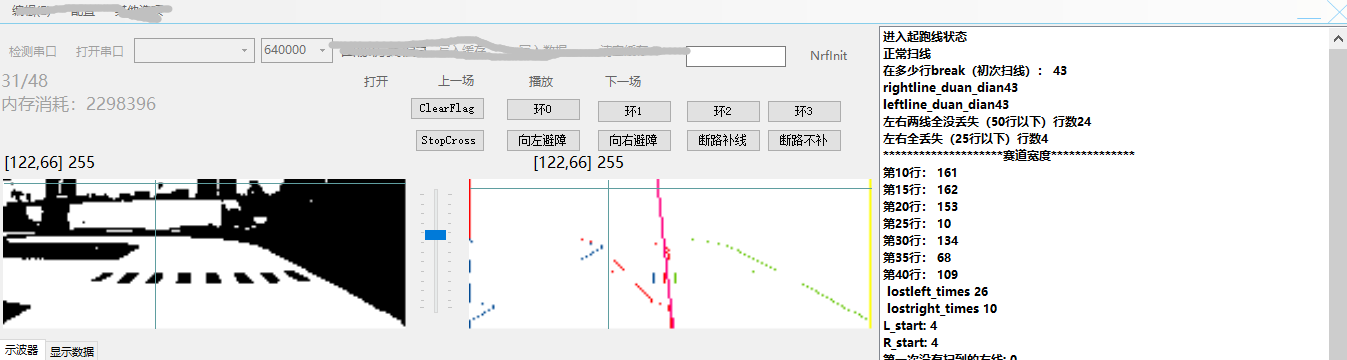进入起跑线状态之后可以进行下一步的特殊操作，如入车库等操作。 这里的行数确定了我们距离起跑线多少能识别出起跑线。当距离较远时，可以发现由于反光，起跑线并不明显。
车库补线
识别车库方向
如何识别车库方位： 我们不是从第0列开始扫的吗？ 设此时已判断为起跑线，我们所扫的那几行的第0列都是黑色，那就是车库在左边。 反之，如果都是白色，那就是车库在右边
车库补线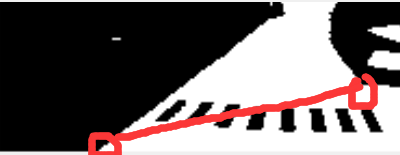如果是检测到为右边车库（已实现），寻找两个端点，然后连接两个端点。 1、 右上拐点 2、 左下拐点
左下拐点： 1、 如果pixels[0,185]为白，往上找，直到pixels[y1,185]为黑（防止姿态的原因导致车偏向道路左边） 2、 从上面确定的第y1行开始往右边扫，扫到的第一个不为黑的点视为左下拐点。
右上拐点： 1、 从第0行往上找，直到pixels[y2,0]为黑 2、 从上面确定的第y2行开始往左边扫，记录下扫到的第一个为白的点的列坐标 3、 遍历[y2, y2+5],选出6行中列坐标最大（最靠左）的列坐标以及那时的行坐标，作为左上拐点
#region[右车库]
//前一个是行坐标，后一个是列坐标
int[] right_garage_left_turn_down = new int;
int[] right_garage_right_turn_up = new int;
void check_guaidian()
{
//首先找左下拐点
byte y1 = 0;
byte x1 = 0;
byte x = 0;
byte y = 0;
// 1、	如果pixels[0, 185]为白，往上找，直到pixels[y1, 185]为黑（防止姿态的原因导致车偏向道路左边）
if (Pixels[0, 185] != 0)   //不为黑,即为白
{

for (y = 1; y < 70; y++)
{
if (Pixels[y, 185] == 0)
{
y1 = y;
break;
}
}
}
else
{
y1 = 0;
}
//2、	从上面确定的第y1行开始往右边扫，扫到的第一个不为黑的点视为左下拐点。

for (x = 185;x>1;x--)
{
if (Pixels[y1, x] != 0)
{
x1 = x;
break;
}
}
//赋值操作
right_garage_left_turn_down = y1;
right_garage_left_turn_down = x1;
//然后找右上拐点
//1、	从第0行往上找，直到pixels[y1,0]为黑
for (y = 0; y < 70; y++)
{
if (Pixels[y, 0] == 0)
{
y1 = y;
break;
}
}
//2、	从上面确定的第y1行开始往左边扫，记录下扫到的第一个为白的点的列坐标
for (x = 0; x < 185; x++)
{
if (Pixels[y1, x] != 0)
{
x1 = x;
break;
}
}
//3、	遍历[y2, y2 + 2],选出6行中列坐标最大（最靠左）的列坐标以及那时的行坐标，作为左上拐点
for (y = (byte)(y1 + 1); y <= y1 + 2; y++)
{
byte X=0;
for (x = 0; x < 185; x++)
{
if (Pixels[y1, x] != 0)
{
X = x;
break;
}
}
if (X >= x1)
{
x1 = X;
y1 = y;
}
}
//赋值操作
right_garage_right_turn_up = y1;
right_garage_right_turn_up = x1;

//展示
SetText("车库右上角"+ right_garage_right_turn_up+"  "+ right_garage_right_turn_up);
SetText("车库左下角" + right_garage_left_turn_down + "  " + right_garage_left_turn_down);
//补线程序
byte j = 0;
int delta = right_garage_right_turn_up - right_garage_left_turn_down;
if (delta == 0) delta = 1;
float k = (right_garage_right_turn_up - right_garage_left_turn_down) * 1.0f / delta;
float b = right_garage_left_turn_down;
for (j = (byte)right_garage_left_turn_down; j <= (byte)right_garage_right_turn_up; j++)
{
int jicun = ((int)(k * j + b));
if (jicun >= 185) jicun = 185;
else if (jicun <= 0) jicun = 0;
lefetline[j] = (byte)jicun;
}
}
#endregion

5.11修改
这几天的一些更加完善的代码，不过还是有许多问题：
#region[起跑线]
//> 1、设置一个栈，用来存储黑色元素。
//> 、从此行的第0行开始往左扫
//> 2、如果遇到黑色元素，入栈，
//> 3、如果遇到白色元素，出栈，出栈的同时统计栈中元素个数，如果栈中元素为4—8个（也就是黑胶的宽度），black_blocks++，否则不加。然后将栈中元素清掉
//> 4、如果此行的black_blocks在8个左右，times++
//> 5、遍历18——23行，如果，times在4个左右，则确定为起跑线。
byte flag_starting_line = 0;
byte garage_direction = 0;      //1为左边,2位右边
void check_starting_line(byte start_point, byte end_point)
{
//int[] black_nums_stack = new int;
byte times = 0;
for (byte y = start_point; y <= end_point; y++)
{
byte black_blocks = 0;
byte cursor = 0;    //指向栈顶的游标
for (byte x = 0; x <= 185; x++)
{
if (Pixels[y,x] == 0)
{
if (cursor >= 20)
{
//当黑色元素超过栈长度的操作   break;
}
else
{
cursor++;
}
}
else
{
if (cursor >= 4 && cursor <= 8)
{
black_blocks++;
cursor = 0;
}
else
{
cursor = 0;
}
}
}
if (black_blocks >= 6 && black_blocks <= 9) times++;
}
if (times >= 1)
{
flag_starting_line = 1;
SetText("进入起跑线状态");
}
else
{
flag_starting_line = 0;
}
}
void check_garage_direction(byte start_point, byte end_point)
{
byte times = 0;
if (flag_starting_line == 1)
{
for (int y = start_point; y <= end_point; y++)
{
if (Pixels[y, 0] == 0)
{
times++;
}
}
if (times >= 3 && times <= 7)
{
garage_direction = 1;   //车库在左边
//SetText("车库在左边");
}
else if (times <= 2)
{
garage_direction = 2;   //车库在右边
//SetText("车库在右边");
}
}
SetText("times"+ times);
}
#endregion
#region[右车库]
//前一个是行坐标，后一个是列坐标
int[] right_garage_left_turn_down = new int;
int[] right_garage_right_turn_up = new int;
void check_guaidian()
{
//首先找左下拐点
byte y1 = 0;
byte x1 = 0;
byte x = 0;
byte y = 0;
// 1、	如果pixels[0, 185]为白，往上找（不超过5行），直到pixels[y1, 185]为黑（防止姿态的原因导致车偏向道路左边）
if (Pixels[0, 185] != 0)   //不为黑,即为白
{

for (y = 1; y < 5; y++)
{
if (Pixels[y, 185] == 0)
{
y1 = y;
break;
}
}
}
else
{
y1 = 0;
}
//2、	从上面确定的第y1行开始往右边扫，扫到的第一个不为黑的点视为左下拐点。

for (x = 185;x>1;x--)
{
if (Pixels[y1, x] != 0)
{
x1 = x;
break;
}
}
//赋值操作
right_garage_left_turn_down = y1;
right_garage_left_turn_down = x1;
//然后找右上拐点

if (Pixels[0, 0] == 0 && Pixels[1, 0] == 0 && Pixels[2, 0] == 0 && Pixels[3, 0] == 0 && Pixels[4, 0] == 0)
{
for (y = 5; y < 70; y++)
{
if (Pixels[y, 0] != 0)//为白
{
y1 = y;
break;
}

}
for (y = y1; y < 70; y++)
{
if (Pixels[y, 0] == 0)
{
y1 = y;
break;
}

}
}
else
{
for (y = 10; y < 70; y++)
{
if (Pixels[y, 0] == 0)
{
y1 = y;
break;
}
}
}
//2、	从上面确定的第y1行开始往左边扫，记录下扫到的第一个为白的点的列坐标
for (x = 0; x < 185; x++)
{
if (Pixels[y1, x] != 0)
{
x1 = x;
break;
}
}
byte y_zhengque = 0;
//3、	遍历[y2, y2 + 2],选出6行中列坐标最大（最靠左）的列坐标以及那时的行坐标，作为左上拐点
for (y = (byte)(y1 + 1); y <= y1 + 2; y++)
{
byte X=0;
for (x = 0; x < 185; x++)
{
if (Pixels[y1, x] != 0)
{
X = x;
break;
}
}
if (X >= x1)
{
x1 = X;
y_zhengque = y;
}
}
y1 = y_zhengque;
//赋值操作
right_garage_right_turn_up = y1;
right_garage_right_turn_up = x1;

//展示
SetText("车库右上角"+ right_garage_right_turn_up+"  "+ right_garage_right_turn_up);
SetText("车库左下角" + right_garage_left_turn_down + "  " + right_garage_left_turn_down);
//补线程序
byte j = 0;
int delta = right_garage_right_turn_up - right_garage_left_turn_down;
if (delta == 0) delta = 1;
float k = (right_garage_right_turn_up - right_garage_left_turn_down) * 1.0f / delta;
float b = right_garage_left_turn_down;
for (j = (byte)right_garage_left_turn_down; j <= (byte)right_garage_right_turn_up; j++)
{
int jicun = ((int)(k * j + b));
if (jicun >= 185) jicun = 185;
else if (jicun <= 0) jicun = 0;
lefetline[j] = (byte)jicun;
}
}
#endregion
#region[左车库]
//前一个是行坐标，后一个是列坐标
int[] left_garage_left_turn_up = new int;
int[] left_garage_right_turn_down = new int;
void check_guaidian1()
{
//首先找右下拐点
byte y1 = 0;
byte x1 = 0;
byte x = 0;
byte y = 0;
// 1、	如果pixels[0, 0]为白，往上找(不可超过5)，直到pixels[y1, 0]为黑（防止姿态的原因导致车偏向道路右边）
if (Pixels[0, 0] != 0)   //不为黑,即为白
{

for (y = 1; y < 5; y++)
{
if (Pixels[y, 0] == 0)
{
y1 = y;
break;
}
}
}
else
{
y1 = 0;
}
//2、	从上面确定的第y1行开始往左边扫，扫到的第一个不为黑的点视为右下拐点。

for (x = 0; x <185; x++)
{
if (Pixels[y1, x] != 0)
{
x1 = x;
break;
}
}
//赋值操作
left_garage_right_turn_down = y1;
left_garage_right_turn_down = x1;
//然后找左上拐点
//如果前5行为黑，则第二次为黑的行数

//否则从第10行往上找
//1、	从第10行往上找，直到pixels[y1,185]为黑
if (Pixels[0, 185] == 0 && Pixels[1, 185] == 0 && Pixels[2, 185] == 0 && Pixels[3, 185] == 0 && Pixels[4, 185] == 0)
{
for (y = 5; y < 70; y++)
{
if (Pixels[y, 185] != 0)//为白
{
y1 = y;
break;
}

}
for (y = y1; y < 70; y++)
{
if (Pixels[y, 185] == 0)
{
y1 = y;
break;
}

}
}
else
{
for (y = 10; y < 70; y++)
{
if (Pixels[y, 185] == 0)
{
y1 = y;
break;
}
}
}
SetText("左上行："+y1);
//2、	从上面确定的第y1行开始往右边扫，记录下扫到的第一个为白的点的列坐标
for (x = 185; x > 1; x--)
{
if (Pixels[y1, x] != 0)
{
x1 = x;
break;
}
}
SetText("y1是" + y1);
//3、	遍历[y2, y2 + 2],选出3行中列坐标最大（最靠左）的列坐标以及那时的行坐标，作为左上拐点
byte y_zhengque = 0;
for (y = (byte)(y1 + 1); y <= (byte)(y1 + 3); y++)
{
byte X = 0;
for (x = 185; x > 1; x--)
{
if (Pixels[y1, x] != 0)
{
X = x;
break;
}
}
if (X <= x1)
{
x1 = X;
y_zhengque = y;
}
}
y1 = y_zhengque;
SetText("y1是"+y1);
//赋值操作
left_garage_left_turn_up = y1;
left_garage_left_turn_up = x1;

//展示
SetText("车库左上角" + left_garage_left_turn_up + "  " + left_garage_left_turn_up);
SetText("车库右下角" + left_garage_right_turn_down + "  " + left_garage_right_turn_down);
//补线程序
byte j = 0;
int delta = left_garage_left_turn_up - left_garage_right_turn_down;
if (delta == 0) delta = 1;
float k = (left_garage_left_turn_up - left_garage_right_turn_down) * 1.0f / delta;
float b = left_garage_right_turn_down;
for (j = (byte)left_garage_right_turn_down; j <= (byte)left_garage_left_turn_up; j++)
{
int jicun = ((int)(k * j + b));
if (jicun >= 185) jicun = 185;
else if (jicun <= 0) jicun = 0;
rightline[j] = (byte)jicun;
}
}
#endregion

展开全文数据结构
• 十五届智能车里面添加了车库元素，如下图，计时要求就是需要我们从车库出发开始计时，入库结束计时，不入库没成绩，因此出入库是非常重要的过程 因为我们选择的是电磁车，没有摄像头，所以我选择了线性CCD作为感知...


结合线性CCD出入库
写在前面

写在前面
我写了一篇总的博客，分享了我参加了十五届智能车竞赛的经历哦~还请多多捧场啦 2020第十五届全国大学生智能汽车竞赛——基础四轮组入门看这一篇就够了！
十五届智能车里面添加了车库元素，如下图，计时要求就是需要我们从车库出发开始计时，入库结束计时，不入库没成绩，因此出入库是非常重要的过程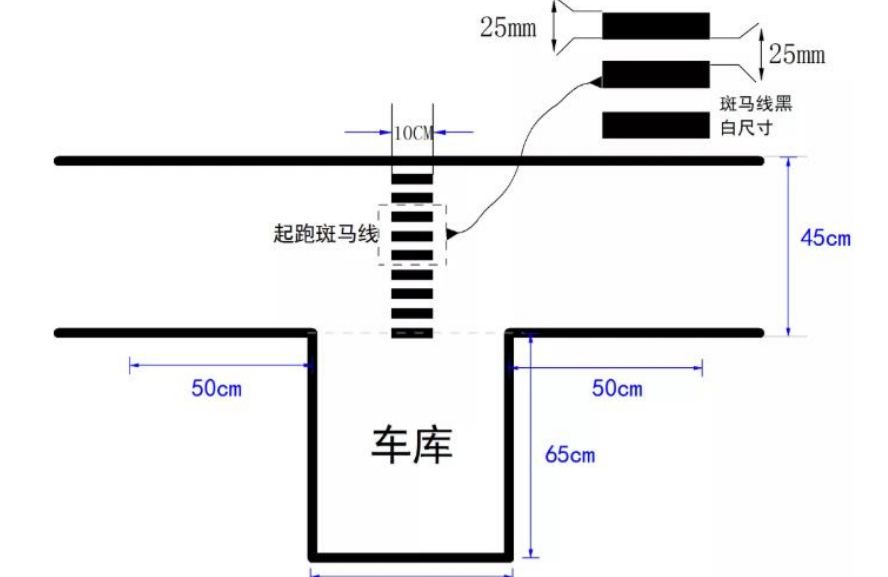因为我们选择的是电磁车，没有摄像头，所以我选择了线性CCD作为感知斑马线的元件，我把他挂在了电磁车的前瞻上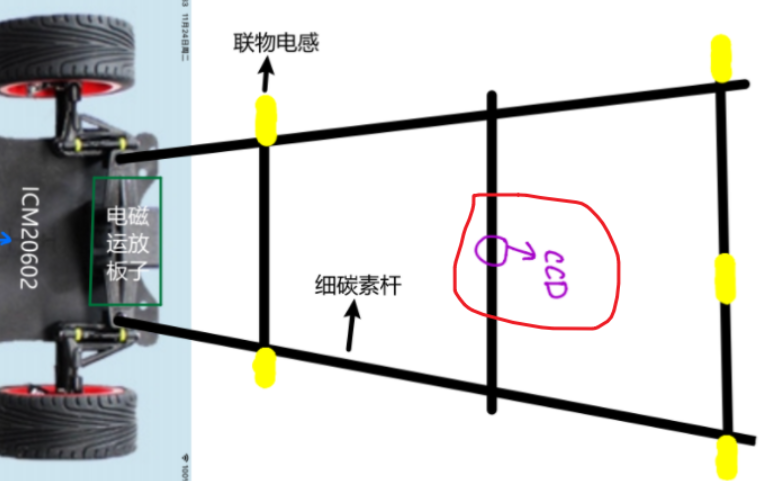我们先来看一下斑马线的CCD图像所以，入库实际上就是需要我们能准确识别上面的那个图像，接下来我就来介绍我的出入库方案
完整链接在我的 古月居博客，传送门在下面：
十六届智能车基础四轮组路标：平稳出库以及线性CCD稳定入库展开全文嵌入式 算法 c语言 图像处理
•研究论文
• 这是一个关于用STM32芯片控制直流电机的一个小程序stm32
• 关于第十五届全国大学生智能汽车竞速比赛的车库元素的一些处理方式


目录
车库元素一览入车库20cm摄像头拍下的车库图起跑线的识别入车库的拉线
出车库

车库元素一览

车库元素是今年赛道元素新增的元素。车库赛道元素是在起跑线旁设置的方形区域，它的尺寸如下图所示：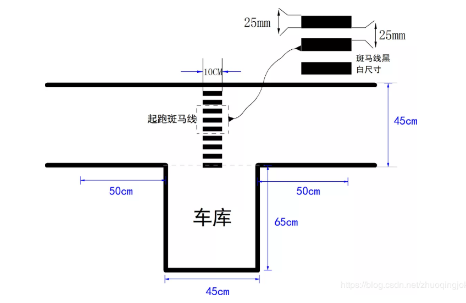车模出发是从车库驶出，在运行一周之后在驶入车库内。比赛时间是从车模驶出车库和返回车库之间的时间差计算。因此计时线圈是放置在车库门口处。赛道上的斑马线和斑马线下的磁铁放置与往届规则相同。 车库应用于“基础四轮组”，“直立节能组”，“双车接力组”。

入车库
20cm摄像头拍下的车库图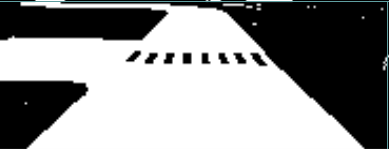起跑线的识别
因为时间紧迫，这里采用最简单的入车库（左入）斑马线识别方法：
找到左前边线的拐点从下（拐点的y坐标）向上（拐点的y坐标+m），从左（拐点的x坐标）到右（中线位置），进行寻找由黑到白的转变边界像素点。找到了第一个边界，以固定的y坐标（转变边界像素点的y坐标+n），从左到右，重新扫线。找到第一个由白转黑的像素点和第一个由黑转白的像素点，记录距离（length），作为判断之后斑马线长度的依据持续向右扫线，每找到一个疑似斑马线的像素块，就把边界像素的距离与length进行比较，在设定误差之内，判定斑马线。记录斑马线数量，与判定起跑线的斑马线数量阈值比较。
代码：
byte Garage_Judge(byte i)
{
byte y = 0, x = 0, count = 0, pixel_cnt = 0, zebraWidth = 0;
//左边车库
if (i == 0)
{
for (OlRow = LeftLine_1.Agl_Row; OlRow <= LeftLine_1.Agl_Row + 20; OlRow++)
{
for (OlLine = (byte)(LeftLine_1.Agl_Line - 5); OlLine >= 93; OlLine--)
{
//SetText_1("Zerba Scan :"+ OlRow+" "+ OlLine);
if (J_Pixels[OlRow][OlLine] == white && J_Pixels[OlRow][OlLine - 1] == black)
{
y = (byte)(OlRow + 3);
//x = (byte)(OlLine-1);
SetText_1("Find Zebra Point: " + y + " " + OlLine);
break;
}
}
if (y != 0)
break;
}
if (y == 0)
{
SetText_1("Lost Zebra Point");
return 0;
}
for (x = LeftLine_1.Agl_Line; x >= 185 - LeftLine_1.Agl_Line; x--)
{
if (J_Pixels[y][x + 1] == white && J_Pixels[y][x] == black)
{
//SetText_1("Zebra Scan Start: " + y + " " + x);
pixel_cnt = 1;
}
else if (J_Pixels[y][x + 1] == black && J_Pixels[y][x] == black)
{
//SetText_1("Zebra Add: " + y + " " + x);
pixel_cnt++;
}
else if (J_Pixels[y][x + 1] == black && J_Pixels[y][x] == white)
{
if (count == 0 && pixel_cnt >= 3 && pixel_cnt <= 10)
{
zebraWidth = pixel_cnt;
SetText_1("zebraWidth = " + zebraWidth);
SetText_1("zebraStart Point: " + x + " " + y);
count = 1;
}
else if (count > 0 && my_fabs(pixel_cnt - zebraWidth) <= 4)
{
//SetText_1("Zebra End: " + y + " " + (x - 1));
count++;
}
else
{
// SetText_1("Zebra Error: " + y + " " + (x - 1));
}
}
}
SetText_1("Zebra Count = " + count);
if (count >= 6)
{
SetText_1("Proved to be Garage");
return 1;
}
else
{
SetText_1("Not Proved to be Garage");
return 0;
}
}
else  //右边车库
{
for (OlRow = RightLine_1.Agl_Row; OlRow <= RightLine_1.Agl_Row + 20; OlRow++)
{
for (OlLine = (byte)(RightLine_1.Agl_Line + 5); OlLine <= 93; OlLine++)
{
//SetText_1("Zerba Scan :"+ OlRow+" "+ OlLine);
if (J_Pixels[OlRow][OlLine] == black && J_Pixels[OlRow][OlLine - 1] == white)
{
y = (byte)(OlRow + 3);
//x = (byte)(OlLine-1);
SetText_1("Find Zebra Point: " + y + " " + OlLine);
break;
}
}
if (y != 0)
break;
}
if (y == 0)
{
SetText_1("Lost Zebra Point");
return 0;
}
for (x = RightLine_1.Agl_Line; x <= 185 - RightLine_1.Agl_Line; x++)
{
if (J_Pixels[y][x - 1] == white && J_Pixels[y][x] == black)
{
//SetText_1("Zebra Scan Start: " + y + " " + x);
pixel_cnt = 1;
}
else if (J_Pixels[y][x - 1] == black && J_Pixels[y][x] == black)
{
//SetText_1("Zebra Add: " + y + " " + x);
pixel_cnt++;
}
else if (J_Pixels[y][x - 1] == black && J_Pixels[y][x] == white)
{
if (count == 0 && pixel_cnt >= 3 && pixel_cnt <= 10)
{
zebraWidth = pixel_cnt;
SetText_1("zebraWidth = " + zebraWidth);
SetText_1("zebraStart Point: " + x + " " + y);
count = 1;
}
else if (count > 0 && my_fabs(pixel_cnt - zebraWidth) <= 4)
{
//SetText_1("Zebra End: " + y + " " + (x - 1));
count++;
}
else
{
// SetText_1("Zebra Error: " + y + " " + (x - 1));
}
}
}
SetText_1("Zebra Count = " + count);
if (count >= 6)
{
SetText_1("Proved to be Garage");
return 1;
}
else
{
SetText_1("Not Proved to be Garage");
return 0;
}
}
}

由于入库图像与环岛入环图像较为相似，入车库判断函数的优先级需要高于入环判断函数。 实践证明，识别成功率很高。
入车库的拉线
拉线分为4个阶段：
阶段0：判断为车库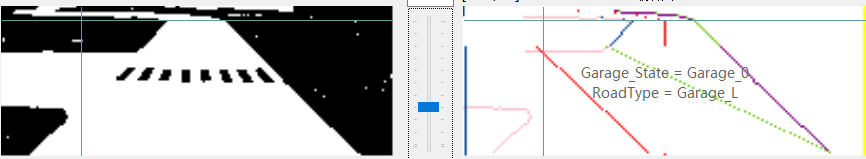阶段1：左前拐点消失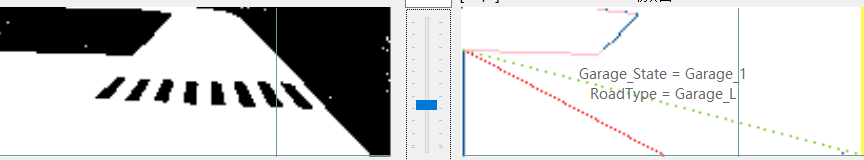阶段2：低行右边边线消失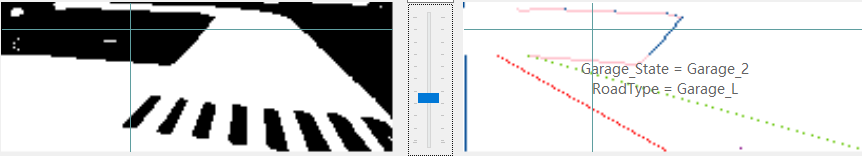阶段3：右边边线重新出现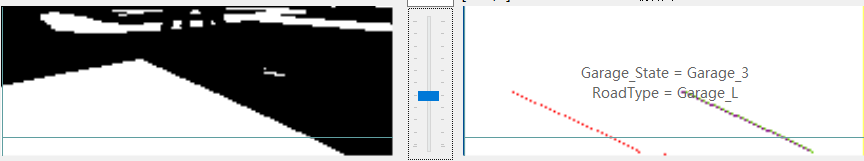阶段4：左边边线出现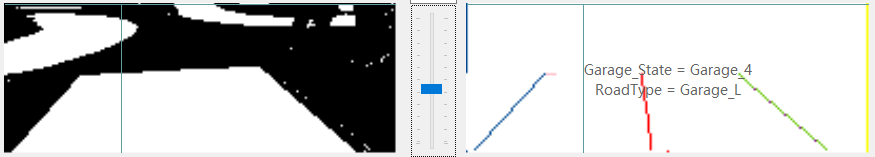出车库
目前最稳定的方法为直接控制出库。
展开全文• 恩智浦 智能车 光电组原理图 驱动电路+k60系统 2018年我自己比赛的电路 实测可用
• 该程序包括寻黑线，避障，寻光，入库，计算路程等。 寻黑线用188，避障和寻光用光敏电阻。
• 基于STM32的智能小车自动循迹及倒车入库设计.rar
• 自动寻迹智能车涉及到当前高技术领域内的许多先进技术，其中最主要的是传感技术、路径规划和运动控制。本课题是以飞思卡尔智能车竞赛为背景，以单片机作为核心控制单元，以摄像头作为路径识别传感器，以直流电机作为...
• 80C51单片机是一款八位单片机，他的易用性和多功能性受到了广大使用者的好评。这里介绍的是如何用80C51单片机来实现长春工业大学的毕业设计，该设计是结合科研项目而确定的设计类课题。本系统以设计题目的要求为目的...
• 三套平衡直立组智能车程序 （2013年K60智能车平衡组国赛代码 、K60_Car、第八届电磁程序）
• 此文件包含完整的代码，只需根据个人车模的不同调整其中中间参数即可，很方便的。。 此文件包含完整的代码，只需根据个人车模的不同调整其中中间参数即可，很方便的。。
• 作者：Sumjess   本次博客内容：   该初始化函数下有以下语句： 一、key_init(KEY_MAX);...用在山外的lcd上，直接调用山外的库函数，该函数内部并无修改，截图如下 ...舵机频率为：SD-5 300hz ...
作者：Sumjess

本次博客内容：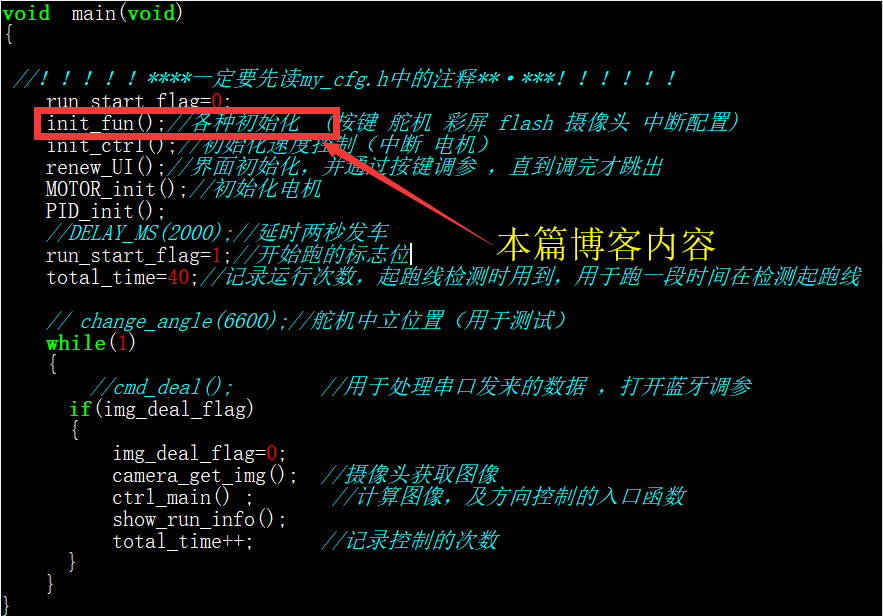该初始化函数下有以下语句：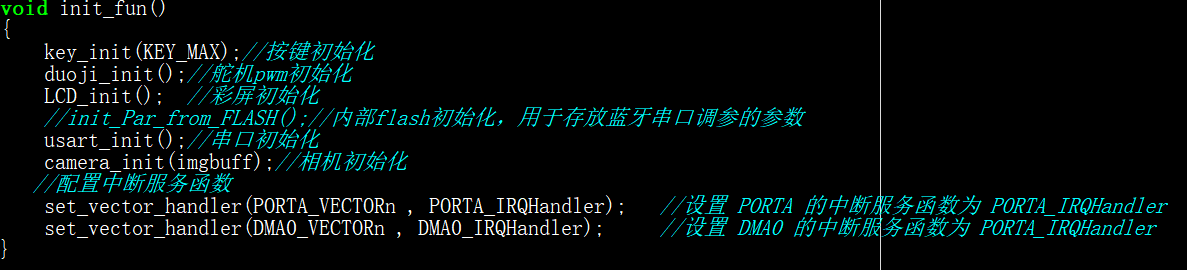一、key_init(KEY_MAX);  ---  按键初始化：
用在山外的lcd上，直接调用山外的库函数，该函数内部并无修改，截图如下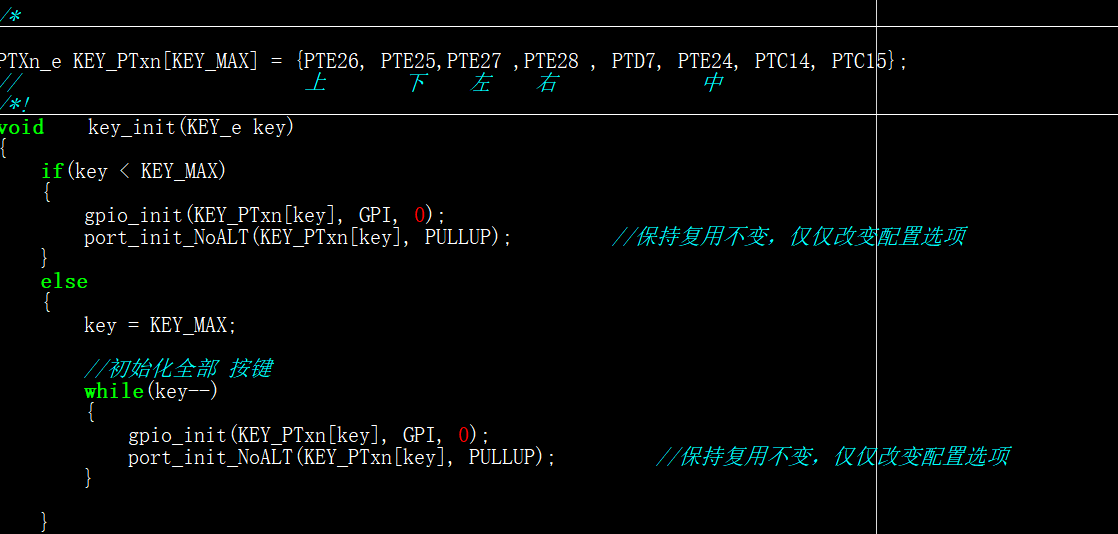二、duoji_init();  ---  舵机初始化：
舵机使用的引脚为：FTM3_CH0_PIN    PTE5
舵机频率为：SD-5  300hz      S3010   50-60hz
舵机打脚中心值、最左最右打脚值为：车膜装好后，写个按键舵机测量程序即可。
精度设置为：70000u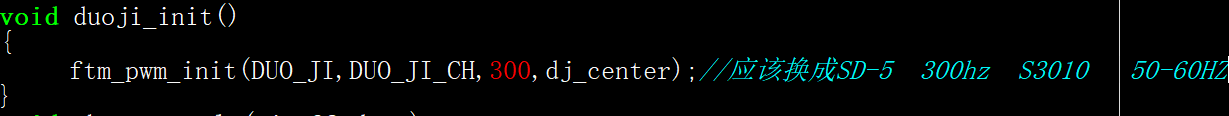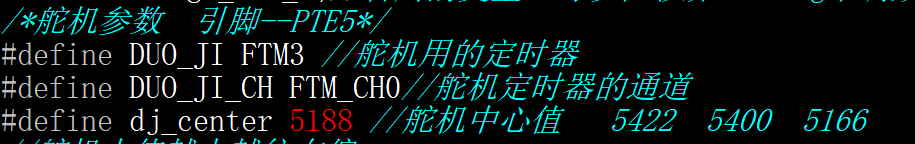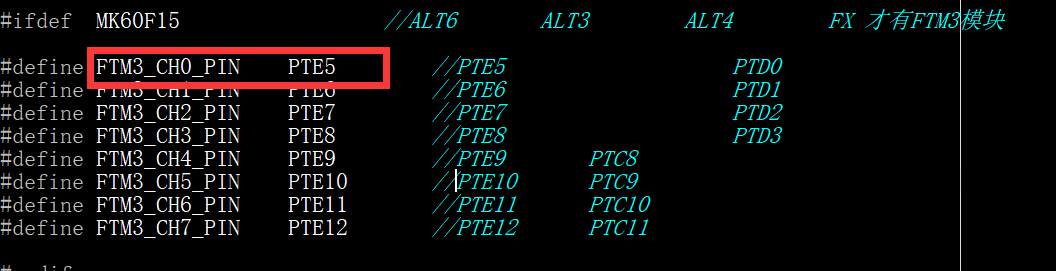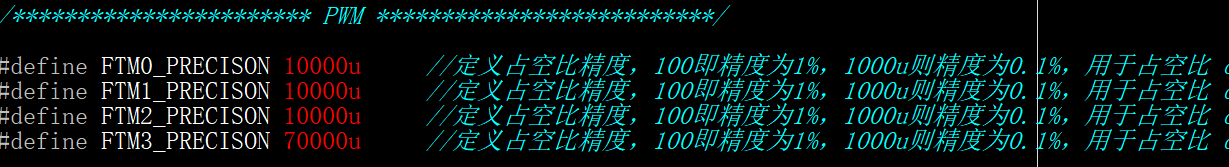三、LCD_init();  ---  LCD初始化：
山外的lcd直接调用山外的库函数，该函数内部并无修改，截图如下：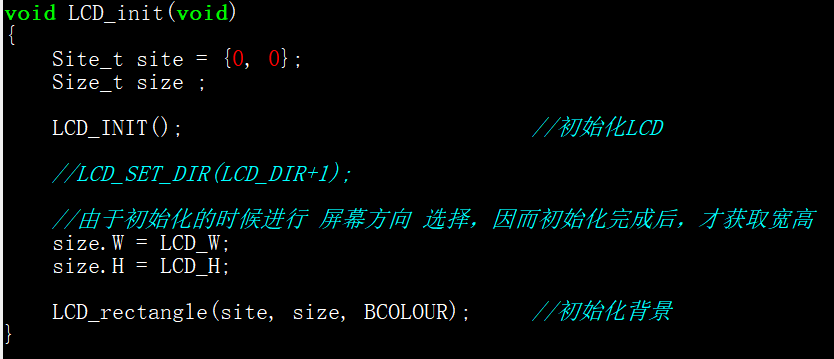注意：如果使用的山外小屏幕，这个地方注意修改：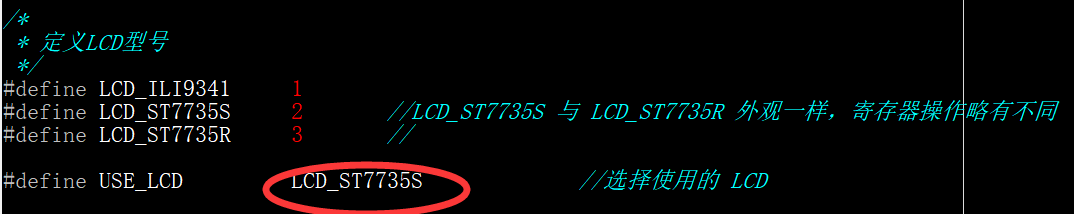四、 usart_init();  ---  串口初始化：
此部分暂时不解释，否则没有连贯性。
五、 camera_init(imgbuff); ---  鹰眼ov7725初始化：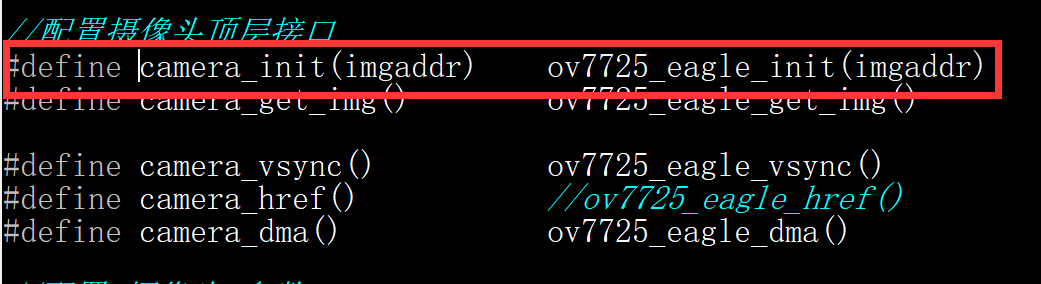引脚和寄存器的初始化：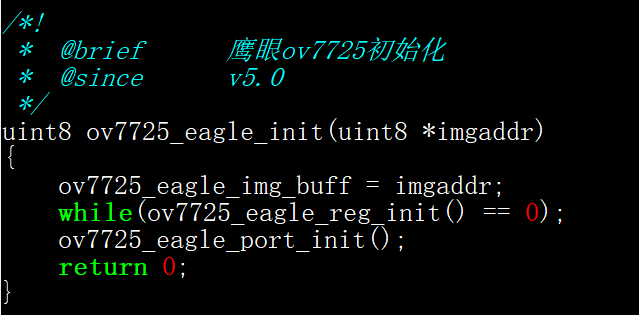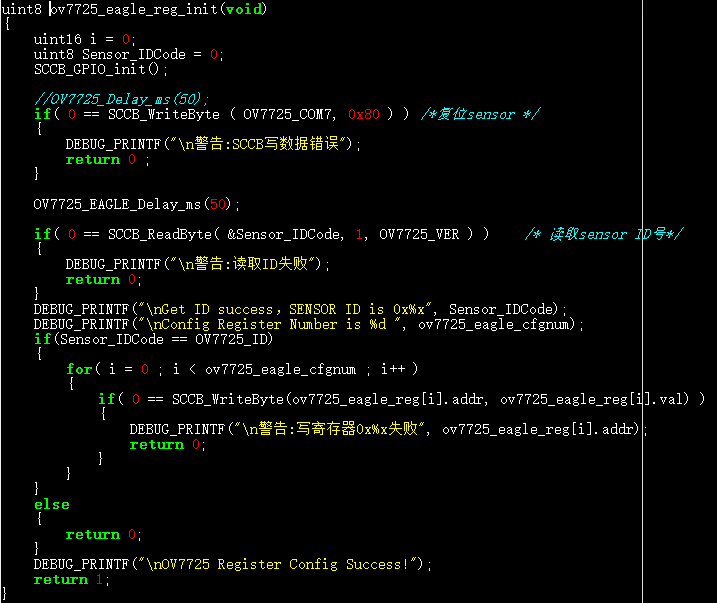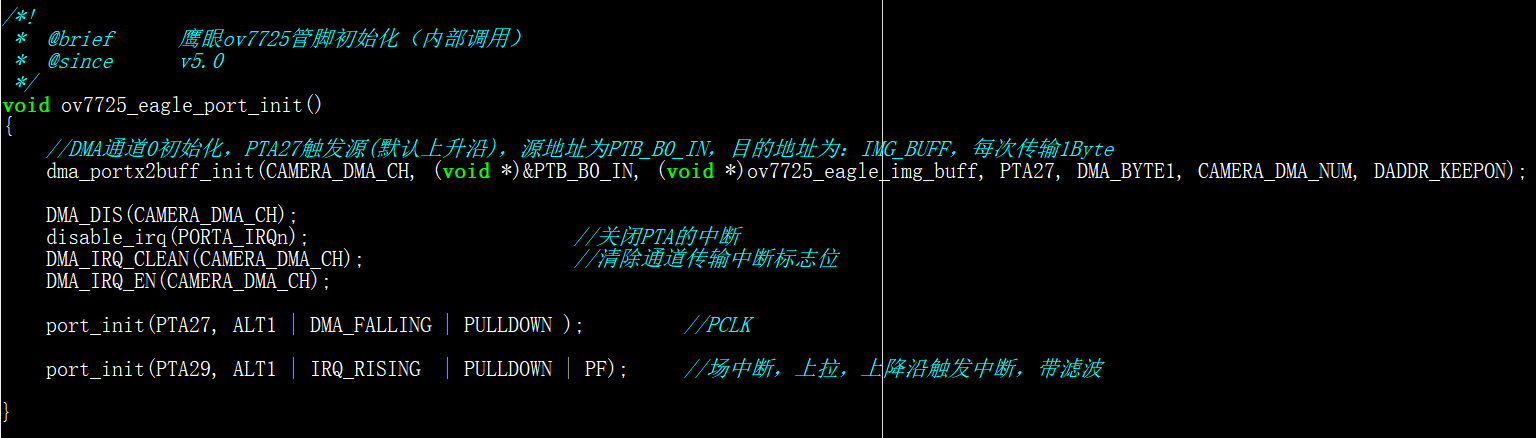五、 配置中断服务函数
① set_vector_handler(PORTA_VECTORn ,PORTA_IRQHandler);
---  设置 PORTA 的中断服务函数为 PORTA_IRQHandler
② set_vector_handler(DMA0_VECTORn , DMA0_IRQHandler);           ---  设置 DMA0 的中断服务函数为 PORTA_IRQHandler
使用DMA采集的原因：
① DMA就是为了尽可能节省CPU资源地把IO状态写进内存。
② DMA只是采集数据的一种方式，它不需要CPU的干预，适合处理信息较多的数据。
什么是场中断、行中断：
摄像头采集数据是一行一行采集的，当采集完一行的图像之后就触发行中断信号，比如360*240的像素，总的240行，当240行全部采集完了之后就触发场中断，所谓的场就是整张图像全部采集。
什么是分辨率、像素：
分辨率就是指像素的多少，像素就是液晶能显示的最小的点。图像都是一个个像素点组成的啊，分辨率 80*60 就是 80列*60行 。

博客更新可能会很慢，请大家耐心等待。

也欢迎各位大佬指正批评！


展开全文• ◎说明: 1 无线射频，手机电路，电视家电，信号处理，电源电路等电路图应有尽有。 2 PCB使用教程，PCB使用技巧，PCB布线规则，PCB layout经验资料丰富精彩。 3 各类电子课件，电子教材，测量仪表，嵌入式技术，制造...
• 随着汽车数量的增加，城市交通状 况日益受到人们的重视，如何进行有效的...拟定的课题名称为基于MATLAB的汽车出入库计时计费系统，带有丰富的人机交互GUI界面。传统的车牌识别，仅仅是对车牌照片进行识别，显得中规中矩
• 作为一名练习时长两年半的练习生，来浅谈下第十五届智能车竞赛规则。 第十五届智能车受疫情影响，终于在千呼万唤中姗姗来迟。细读规则，便会发现今年的智能车和往年有所不同。 今年的组别设置有竞速组4组：基础...
• SmartyPants将ASCII标点字符转换为“智能”印刷标点HTML实体。例如： TYPE ASCII HTML Single backticks 'Isn't this fun?' ‘Isn’t this fun?’ Quotes "Isn't this fun?" ...
• ## 智能车AI电磁部署学习 (一)

千次阅读 多人点赞 2021-02-14 00:52:02
b乎:如何评价2020届智能车比赛的AI电磁组？ 通过学长介绍，在假期观看了吴恩达老师的机器学习与深度学习相关课程。 2 学习基础 以视频为主，笔记为辅。 在每周课程结束后，自己编写课后作业，与CSDN版课后作业和和鲸...机器学习 深度学习 tensorflow python
• 飞思卡尔智能车知识总结 一、摄像头部分 （一）摄像头的选择 鹰眼摄像头：硬件二值化，改变阈值可以改变图像的黑白对比度。阈值越低，图像越白；阈值越高，图像越黑。 神眼摄像头：有红外透视，全局快门，立体机器...
• 另一方面，在未来智能化技术普及之后，人类将更少地参与到泊车 环境中，在条件允许的宽敞情况下，车辆可以适当提高车速，提升泊车效率，但 车速稍做提高，车辆动态响应的稳定性便不可忽视。因此简单的稳态运动假设不...
• 第十五届恩智浦智能汽车竞赛结束了，我们第一回做四轮组的小白，摸爬滚打了一个月，中间有划水，也有熬夜，走了许多弯路，最终成绩很惨，没能完赛，（虽然我自己感觉离完赛就差一个坡道），期间还是学习了很多知识，...单片机 iar
• 随着省赛的落幕，近一年的智能车的生涯也就此画上了句号，虽然结果没有那么耀眼，但也算对得起三个大一萌新这么久以来不分昼夜所付出的心血。 在此我想对电磁车中的算法和赛场上一些的状况作进一步总结，也算是给...
• 在此次智能车竞赛中，我选择了摄像头为主，电磁简单辅助路径规划，帮助车身在出弯后能快速回正，只不过后来因为赛场电磁线原因，关闭了电磁辅助，采用了纯摄像头循迹。 关于电磁循迹，我并没有使用归一化，只是简单...
• 回来可以私聊，因为我只做过一次智能车哈，如果遇到我的盲区，我会介绍对应学长给你们去询问。 选队友：   要选择能坚持到最后的，别有些队伍到最后就一个人在搞，宁愿人员不全，我也不要垃圾，因为我如果......